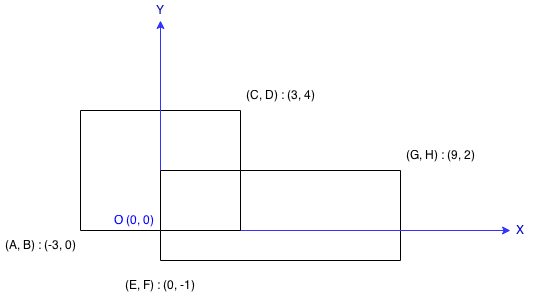# Question

Formatted question description: https://leetcode.ca/all/223.html

# 223. Rectangle Area

Medium

## Description

Find the total area covered by two rectilinear rectangles in a 2D plane.

Each rectangle is defined by its bottom left corner and top right corner as shown in the figure.Example:

Input: A = -3, B = 0, C = 3, D = 4, E = 0, F = -1, G = 9, H = 2

Output: 45

Note:

Assume that the total area is never beyond the maximum possible value of int.

# Algorithm

First find out all the cases of disjoint, there are only four types, one rectangle does not overlap at the top, bottom, left, and right positions of the other. In these four cases, the sum of the area of the two rectangles is returned.

In all other cases, two rectangles have an intersection. At this time, just calculate the length and width to find the size of the intersection area, and then subtract the intersection area from the sum of the two rectangular areas, to get the final answer.

# Code

• 
public class Rectangle_Area {

class Solution {
public int computeArea(int A, int B, int C, int D, int E, int F, int G, int H) {
int erea1 = (C - A) * (D - B), area2 = (H - F) * (G - E);
if (E >= C || F >= D || B >= H || A >= G) {
return erea1 + area2;
} else {
return erea1 + area2 - ((Math.min(G, C) - Math.max(A, E)) * (Math.min(D, H) - Math.max(B, F)));
}
}
}
}

############

class Solution {
public int computeArea(int ax1, int ay1, int ax2, int ay2, int bx1, int by1, int bx2, int by2) {
int a = (ax2 - ax1) * (ay2 - ay1);
int b = (bx2 - bx1) * (by2 - by1);
int width = Math.min(ax2, bx2) - Math.max(ax1, bx1);
int height = Math.min(ay2, by2) - Math.max(ay1, by1);
return a + b - Math.max(height, 0) * Math.max(width, 0);
}
}


• class Solution:
def computeArea(
self,
ax1: int,
ay1: int,
ax2: int,
ay2: int,
bx1: int,
by1: int,
bx2: int,
by2: int,
) -> int:
a = (ax2 - ax1) * (ay2 - ay1)
b = (bx2 - bx1) * (by2 - by1)
width = min(ax2, bx2) - max(ax1, bx1)
height = min(ay2, by2) - max(ay1, by1)
return a + b - max(height, 0) * max(width, 0)

############

class Solution(object):
def computeArea(self, A, B, C, D, E, F, G, H):
"""
:type A: int
:type B: int
:type C: int
:type D: int
:type E: int
:type F: int
:type G: int
:type H: int
:rtype: int
"""
area = (C - A) * (D - B) + (G - E) * (H - F)
overlap = max(min(C, G) - max(A, E), 0) * max(min(D, H) - max(B, F), 0)
return area - overlap


• class Solution {
public:
int computeArea(int ax1, int ay1, int ax2, int ay2, int bx1, int by1, int bx2, int by2) {
int a = (ax2 - ax1) * (ay2 - ay1);
int b = (bx2 - bx1) * (by2 - by1);
int width = min(ax2, bx2) - max(ax1, bx1);
int height = min(ay2, by2) - max(ay1, by1);
return a + b - max(height, 0) * max(width, 0);
}
};

• func computeArea(ax1 int, ay1 int, ax2 int, ay2 int, bx1 int, by1 int, bx2 int, by2 int) int {
a := (ax2 - ax1) * (ay2 - ay1)
b := (bx2 - bx1) * (by2 - by1)
width := min(ax2, bx2) - max(ax1, bx1)
height := min(ay2, by2) - max(ay1, by1)
return a + b - max(height, 0)*max(width, 0)
}

func max(a, b int) int {
if a > b {
return a
}
return b
}

func min(a, b int) int {
if a < b {
return a
}
return b
}


• ﻿// https://leetcode.com/problems/rectangle-area/

using System;

public partial class Solution
{
public int ComputeArea(int A, int B, int C, int D, int E, int F, int G, int H)
{
var area = (long)(C - A) * (D - B) + (G - E) * (H - F);
var overlapA = Math.Max(A, E);
var overlapB = Math.Max(B, F);
var overlapC = Math.Min(C, G);
var overlapD = Math.Min(D, H);
if (overlapA < overlapC && overlapB < overlapD)
{
area -= (overlapC - overlapA) * (overlapD - overlapB);
}
return (int)area;
}
}

• function computeArea(
ax1: number,
ay1: number,
ax2: number,
ay2: number,
bx1: number,
by1: number,
bx2: number,
by2: number,
): number {
const a = (ax2 - ax1) * (ay2 - ay1);
const b = (bx2 - bx1) * (by2 - by1);
const width = Math.min(ax2, bx2) - Math.max(ax1, bx1);
const height = Math.min(ay2, by2) - Math.max(ay1, by1);
return a + b - Math.max(width, 0) * Math.max(height, 0);
}